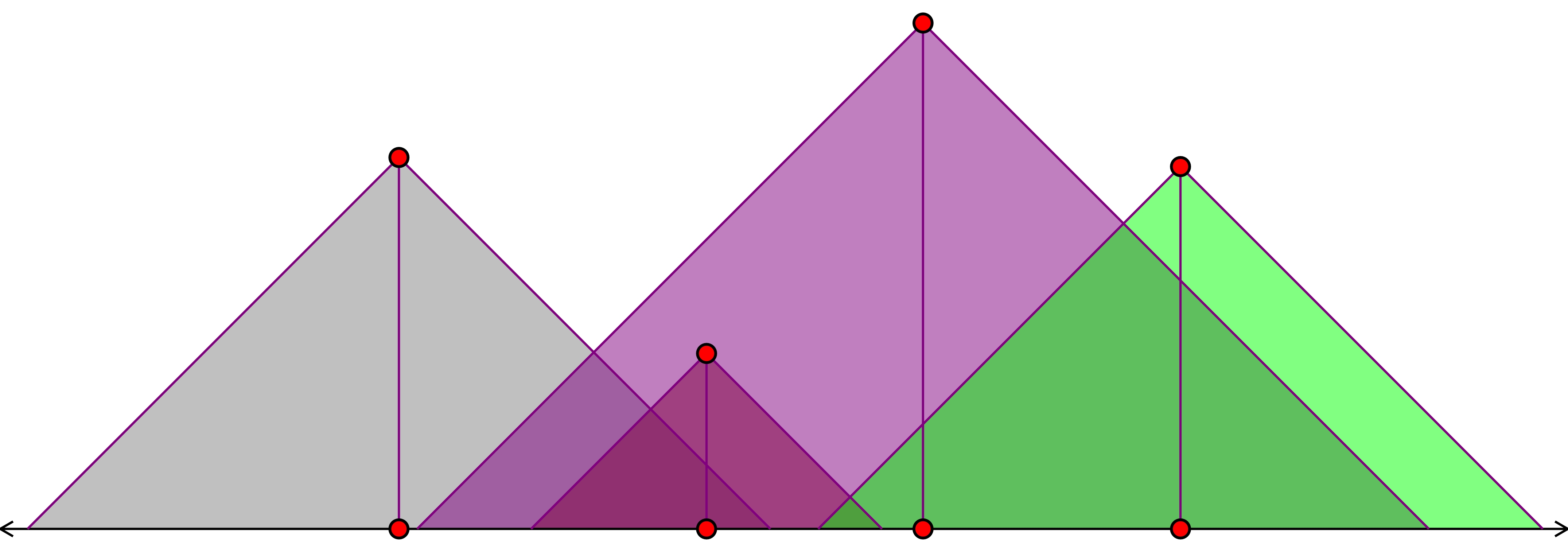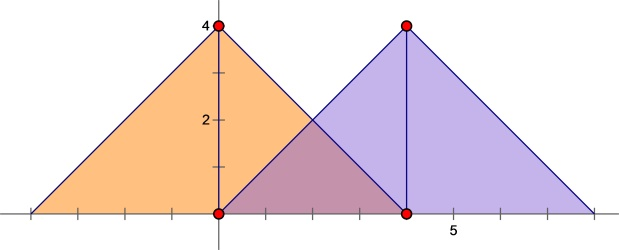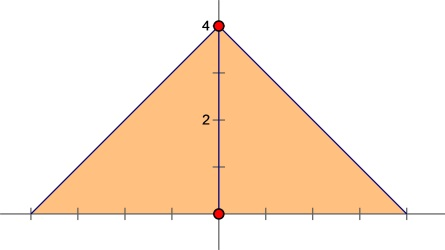# #6213. 「美团 CodeM 决赛」radar

#### 题目描述

Lyra 在玩一款游戏，在这款游戏中，Lyra 要在国境线上安装一列雷达，国境线可以看做一个数轴，第 $i$ 个雷达横坐标在 $x_i$ 的位置，高度最大为 $y_i$。雷达可以向下方至斜下方 $45^\circ$ 角范围内扫描，所以 $(x_0,y_0)$ 点的雷达可以扫描满足 $0 \leq y \leq y_0-|x-x_0|$ 的点组成的区域，若干个雷达的扫描区域是每个雷达扫描点集的并。$i$ 个雷达初始时高度为 $0$，Lyra 可以花费 $c_i$ 元将其高度提升 $1$，注意高度只能是整数。所有雷达布置完之后 Lyra 对于雷达能覆盖到的二维区域，获得等同于其面积的收入。

#### 样例输入

2
2
0 4 1
4 4 3
2
0 4 0
4 100 100

#### 样例输出

12.00
16.00

#### 样例解释#### 数据范围与提示

$T \leq 500$
$1 \leq n \leq 10^5$$\sum n \leq 10^6$
$0 \leq |x_i|,y_i,c_i \leq 10^8$